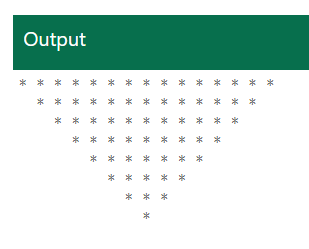# Inverted Pyramid Pattern Algorithm and Example in C#

Following is example of inverted pyramic * pattern with 8 rows:

``* * * * * * * * * * * * * * *   * * * * * * * * * * * * *     * * * * * * * * * * *       * * * * * * * * *         * * * * * * *           * * * * *             * * *               * ``

Concept for achieving algorithm

In the above example - if we inspect we can find that '*' count from botton to up as follows:

1. 8th row=> 1 star,
2. 7th row=> 3 stars,
3. 6th row=> 5 stars,
4. 5th row=> 7 stars,
5. 4th row=> 9 stars,
6. 5th row=> 11 stars,
7. 4th row=> 13 stars,
8. ..
9. ...
10. ...

So it's a odd number series. And odd number series formula will be (2n-1) where n is starting with 1.

Method for generating inverted pyramic pattern

The pattern will be divided into two part:

1. A for loop will be used to print blank spaces
2. A for loop will be used to print odd number series (2n-1)

Algorithm

1. Initialize variables i, j and rows for rows, blank spaces and number of rows respectively.
2. The number of rows here is 8, the user can take any number.
3. Initialize a for loop that will work as the main loop in printing the pattern and drive the other loops inside it.
4. Initialize a for loop that will print the blank spaces inside the main loop.
5. Now, in order to print the star pattern in odd number. To do that, we will initialize a for loop with the condition given as 2 * i – 1, because the number of stars is odd.

Here is example in C#

``using System;namespace ConsoleApp1{    public class Program    {        public static void Main(string[] args)        {            int i, j, rows = 8;            for (i = rows ; i >= 1; i--)            {                // Loop to print the blank spaces                for (j = rows-i ; j >=1; j--)                {                    Console.Write("  ");                }                         // Loop to print the stars                for (j =  (2 * i - 1); j >=1; j--)                {                    Console.Write("* ");                }                         // Move to the next line to complete the pattern                Console.Write("\n");            }         }    }}``You can try online C# editor here: Inverted Pyramid